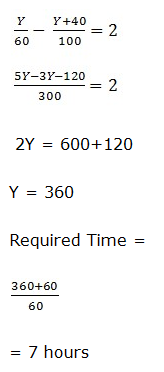# IBPS Clerk Prelims Quantitative Aptitude 2021 (Day-02)

Dear Aspirants, Our IBPS Guide team is providing new series of Quantitative Aptitude Questions for IBPS Clerk Prelims 2021 so the aspirants can practice it on a daily basis. These questions are framed by our skilled experts after understanding your needs thoroughly. Aspirants can practice these new series questions daily to familiarize with the exact exam pattern and make your preparation effective.

Start Quiz

Approximations

Directions (01-05): What approximate value should come in the place of (?) in the following questions.

1) 25.03% of 247.91 – 15.18% of 140.05 = ?

A.41

B.35

C.31

D.46

E.50

2) (82.01 * √50) ÷ ? = 287.08

A.0.5

B.1

C.1.5

D.2

E.2.5

3) (16.51 * 11.98) ÷ 6 = ? + √170

A.21

B.11

C.24

D.28

E.32

4) 959.91 ÷ 24.09 = 37.08 + (? ÷ 3)

A.15

B.12

C.18

D.21

E.9

5) (17.03)2 + (30.98)2 – (8.89)3 = ?

A.521

B.527

C.534

D.541

E.549

SI AND CI

6) If the compound interest on a certain sum for 2 years at 10% per annum is Rs 10500, then the simple interest on the same sum at the same rate for 2 years would be?

A.11500

B.12500

C.10000

D.10050

E.None of these

Mensuration

7) The radius of the semicircle is equal to half the side of a square and the area of a square is 784 sq. cm. Then, find the perimeter of a semicircle?

A.48 cm

B. 64 cm

C.72 cm

D.96 cm

E.None of these

Ages

8) The sum of the present ages of Jatin, Piyush and Suraj is 160 years. 16 years ago, the ratio of their ages was 1:3:4. What is the present age of Suraj?

A.70 years

B.56 years

C.72 years

D.68 years

E.None of these

Time, speed and dictance

9) The time taken to travel a distance of Y km at the speed of 60 kmph is 2 hours more than the time taken to travel (Y+40) km at the speed of 100 kmph. Find the time taken to travel (Y+60) km at the speed of 60 kmph.

A.4 hours

B.10 hours

C.5 hours

D.7 hours

E.None of these

Number system

10) There are three numbers in the ratio 5:10:19. The sum of the largest and the smallest number is 140 more than the second largest number. What is the smallest number?

A.190

B.100

C.45

D.50

E.None of these

25.03% of 247.91 – 15.18% of 140.05 = ?

62 – 21 = ?

? = 41

(82.01 * √50) ÷ ? = 287.08

574 /? = 287

? = 2

(16.51 * 11.98) ÷ 6 = ? + √170

204/6 = ? + 13

? = 21

959.91 ÷ 24.09 = 37.08 + (? ÷ 3)

40 – 37 = ?/3

? = 9

(17.03)2 + (30.98)2 – (8.89)3 = ?

289 + 961 – 729 = ?

? = 521

Compound Interest = Rs 10500

P*((1+10/100)2-1) = Rs 10500

P(1.21-1) = Rs 10500

P= 10500/0.21

P = Rs 50,000

SI = 50000*10*2/100

SI = Rs 10,000

(Or)

CI at 10% pa for 2 years =

= 21%

21% = 10500

SI at 10% for 2 years is equivalent to 10 x 2 = 20%

20% = 10500/21 x 20 = 10000

Side of the square = √784=28 cm

Perimeter of the semicircle = πr+2r

= (22/7 x 14+2 x 14)= 44+28=72 cm

Jatin + Piyush + Suraj = 160

Now, 16 years ago,

Jatin -16 : Piyush -16 : Suraj – 16 = 1:3:4

160 – 48 = 112

Suraj’s age 16 years ago =  112 x 4/8=56

Suraj’s present age = 56 + 16 = 72 years

According to question,Let the number be 5X, 10X and 19X.

According to question,

5X + 19X – 10X = 140

24X -10X = 140

14X = 140

X = 10

The smallest number = 5 x 10 = 50